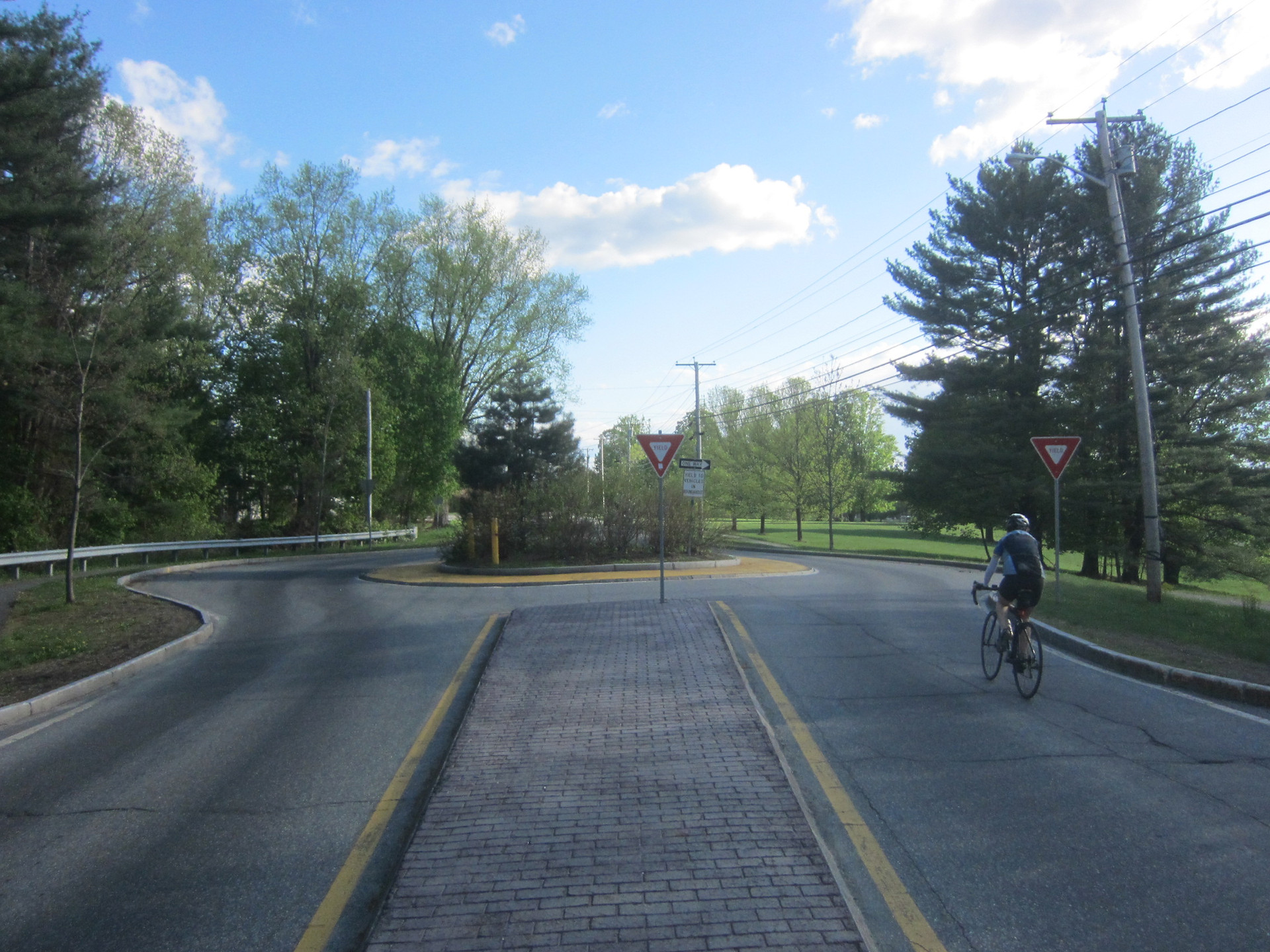###### due Thursday October 01 5:00 pm##### Question 1
###### ​

The trip production for the three TAZs in a city is given as well as the attractiveness of each TAZ is given in Table 1.

TAZ 1: Production = 500, Attractiveness = 2

TAZ 2:Production = 1500, Attractiveness = 5

TAZ 3:Production = 400, Attractiveness = 2

The inter-zonal impedance is given in Table 2.

1 to 1:   Impedance = 1.0

1 to 2:  Impedance = 5.0

1 to 3:  Impedance = 2.0

2 to 1:  Impedance = 6.0

2 to 2: Impedance = 2.5

2 to 3: Impedance = 4.0

3 to 1:  Impedance = 2.0

3 to 2: Impedance = 3.5

3 to 3: Impedance = 0.5

The friction factor is given as 1/(wij)2.  (That is 1 over w squared) or (the calibration is 2)

The socio-economic factor is equal to 1.

Based on the information above, is this a city with mixed land-use?  Explain.

Calculate the trip interchange between each TAZs.

##### Question 2
###### ​

Which of the four steps of the four step process is needed to estimate how many new trips would be produced if an addition to Storrs Center was made that doubled the size of the center?

Which of the four steps would be used to determine what percentage of car trips between Storrs to Hartford would use a specific route?

##### Question 3
###### ​

uk= ak– 0.025 X1– 0.032 X2– 0.015 X3– 0.002 X4

where

ak - model constant

x1 – access plus egress time (min)

x2 – waiting time (min)

x3 – line haul time (min)

x4 – out of pocket cost (dollars)

Car:    a = -0.60    x1=10    x2=0      x3=200    x4=40

Rail:   a = +0.30    x1=20    x2=25   x3=220     x4=60

Air:     a = -0.30    x1=60    x2=75   x3=40       x4=55